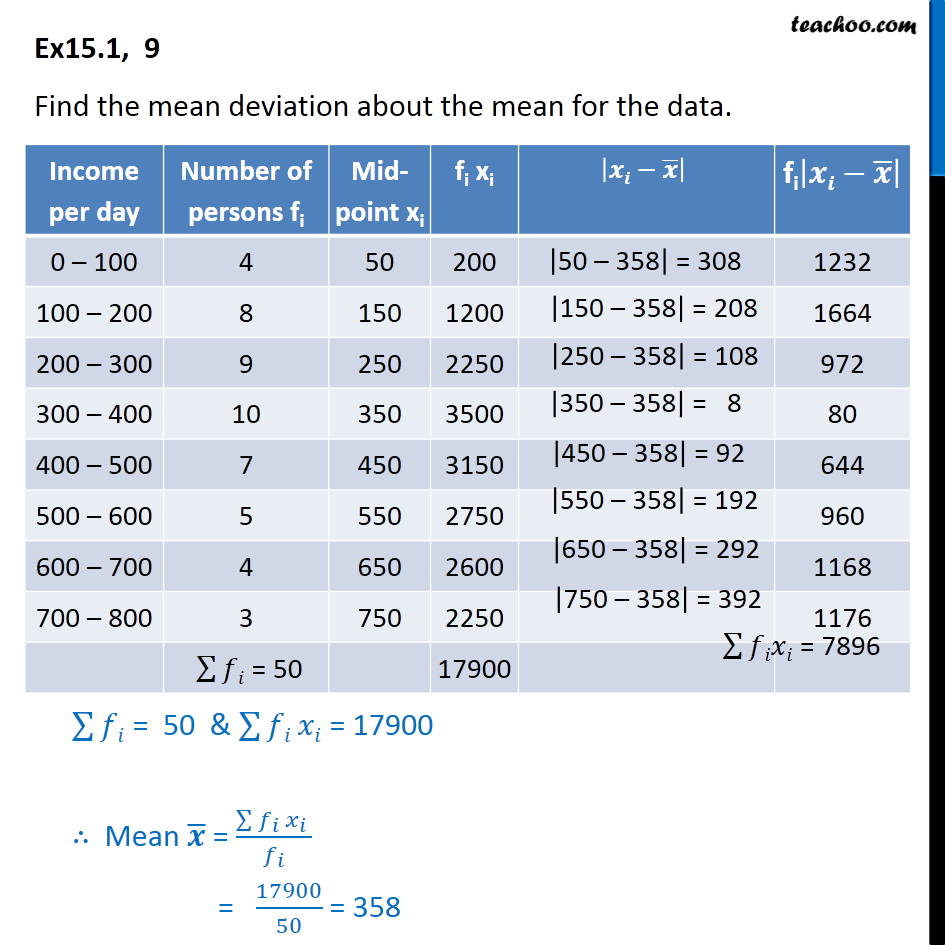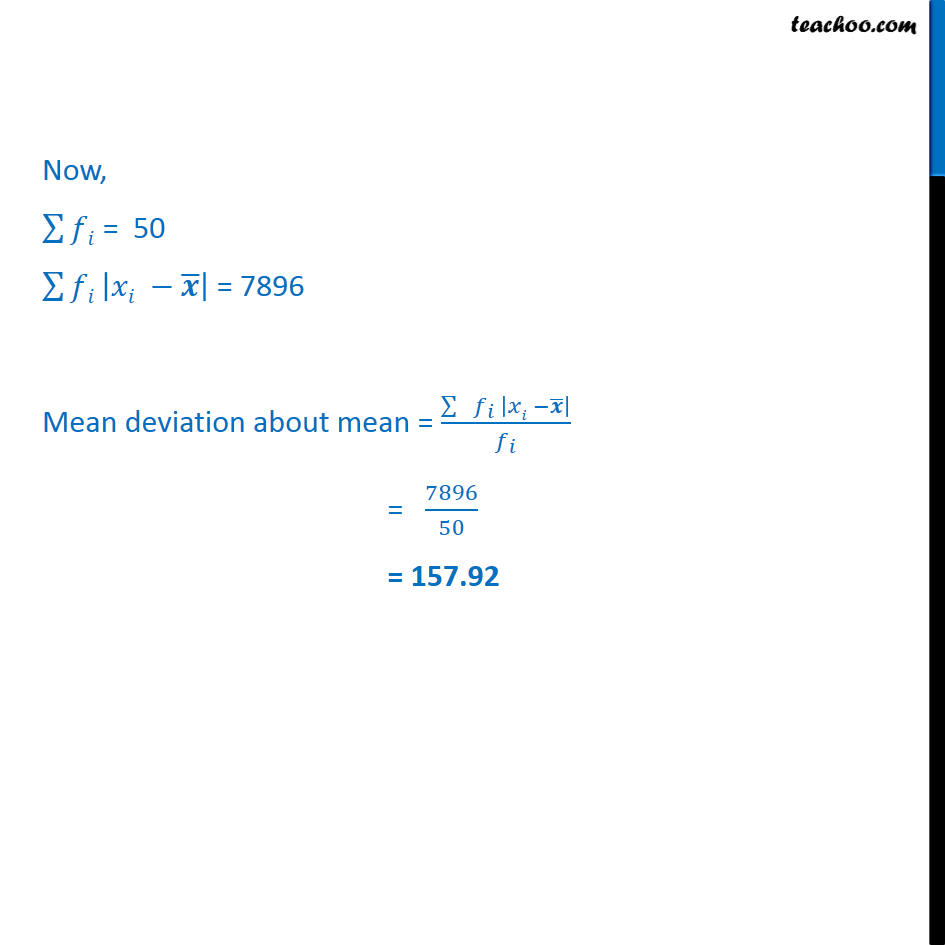Ex 13.1

Chapter 13 Class 11 Statistics
Serial order wiseLearn in your speed, with individual attention - Teachoo Maths 1-on-1 Class

### Transcript

Ex 13.1, 9 Find the mean deviation about the mean for the data. 128 = 50 & 128 = 17900 Mean = ( _ _ )/ _ = 17900/50 = 358 Now, 128 = 50 128 | | = 7896 Mean deviation about mean = ( _ | | )/ _ = 7896/50 = 157.92